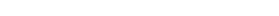# Isosceles Triangles on the GMAT

The GMAT quantitative section asks, among other things, about geometry. One of the GMAT’s favorite figures is the isosceles triangle.  An isosceles triangle is one that has two congruent sides.  Knowing simply that about a triangle has profound implications for answer GMAT Problem Solving & Data Sufficiency questions.

## Euclid’s Remarkable Theorem

Euclid first proved this theorem over 2200 years ago.  The theorem says:

If the two sides are equal, then the opposite angles are equal

and

if the two angles are equal, then the opposite sides are equal.

It can be used for deductions in both directions (logically, this is called a “biconditional” statement”).  Another way to say this: a statement about equal angles is sufficient to conclude equal sides; conversely, a statement about equal sides is sufficient to conclude equal angles.  Remember that on GMAT Data Sufficiency!## Isosceles Triangles and the 180º Triangle Theorem

Another favorite GMAT geometry fact is that the sum of all three angles in a triangle − any triangle − is 180º.  This is particularly fruitful if combined with the Isosceles Triangle Theorem.

Suppose you are told that Triangle ABC is isosceles, and one of the bottom equal angles (called a “base angle”) is 50º.  Then immediately you know that the measure of the other base angle is also 50º, and that means the top angle (the “vertex angle”) must be 80º.  Knowing the measure of one base angle is sufficient to find the measures of all three angles of an isosceles triangle.

Suppose you are told that Triangle ABC is isosceles, and the vertex angle is 50º.  Well, you don’t know the measures of the base angle, but you know they’re equal. Let x be the degrees of the base angle; then.  So, each base angle is 65º.  Knowing the measure of the vertex angle is sufficient to find the measures of all three angles of an isosceles triangle.

BUT, if you are told that Triangle ABC is isosceles, and one of angles is 50º, but you don’t know whether that 50º is a base angle or a vertex angle, then you cannot conclude anything about the other angles in the isosceles triangle without more information.  That’s a subtle but important distinction to remember on GMAT Data Sufficiency.

## Free Practice Questions on Triangles

http://gmat.magoosh.com/questions/1019

http://gmat.magoosh.com/questions/1024

## Author

•Mike served as a GMAT Expert at Magoosh, helping create hundreds of lesson videos and practice questions to help guide GMAT students to success. He was also featured as "member of the month" for over two years at GMAT Club. Mike holds an A.B. in Physics (graduating magna cum laude) and an M.T.S. in Religions of the World, both from Harvard. Beyond standardized testing, Mike has over 20 years of both private and public high school teaching experience specializing in math and physics. In his free time, Mike likes smashing foosballs into orbit, and despite having no obvious cranial deficiency, he insists on rooting for the NY Mets. Learn more about the GMAT through Mike's Youtube video explanations and resources like What is a Good GMAT Score? and the GMAT Diagnostic Test.